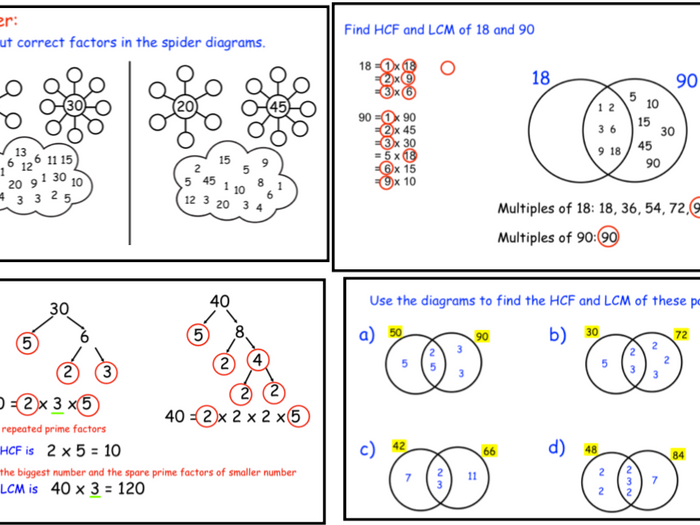2/3 Lessons.
Objectives:

1. Be able to write a number as a product of its prime factors.
2. Be able to construct a tree diagram to find the prime factors of a number.
3. Be able to calculate the HCF and LCM of two numbers using Venn diagrams.
4. Be able to find the HCF and LCM of two numbers when given the prime factorisation of each numbers.
Includes:
Lesson starters;
Worked examples;
Practise questions;
Plenary questions.
For more resources go to www.themathsgeezer.co.uk
\$5.23
Save for later

### Info

Created: Sep 26, 2018

Updated: May 20, 2019

pptx, 5 MB

KS3-4_Number_Prime-factors_Prime-factorisation_HCF-LCM-copy

Report a problem